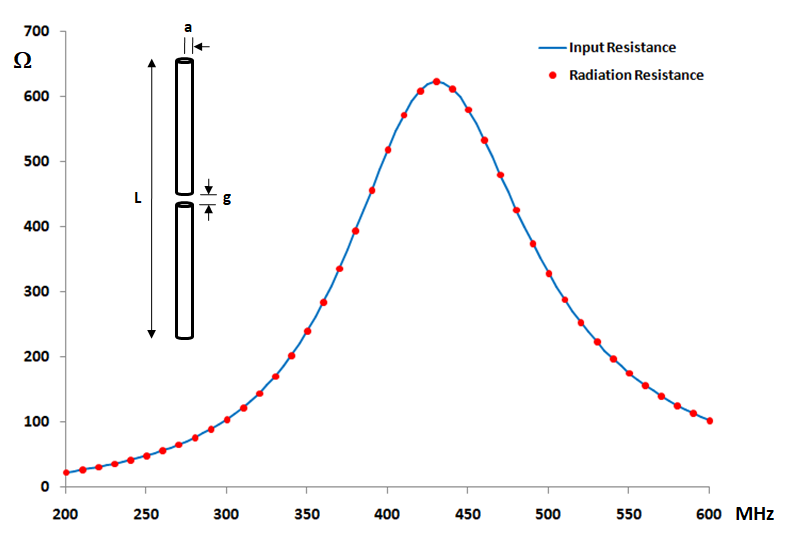How can we help?

# Dipole Gain and Radiation Resistance

In absence of power losses, the power radiated by any antenna must be equal to its input power. In other words, the radiation resistance of a lossless antenna must match its input resistance. When comparing these two resistances we have another validation check of a numerical method.

Fig. 1 shows the input resistance of a center-fed dipole antenna of length L = 0.5 m, radius a = 5 mm and source gap g = L/200 as a function of frequency. On the other hand, the radiation resistance has been calculated as the ratio of the radiated power to the square of the absolute value of the input current at the source position.Fig. 1: Input and radiation resistances of a lossless center-fed cylindrical antenna as a function of frequency for L = 0.5 m, a = 5 mm and g = L/200.

The radiated power can be obtained from the Power Budget > window in the AN-SOF Results menu. The difference between both resistances is numerically negligible as can be seen. In Fig. 2 the percentage difference between the input and radiation resistances plotted in Fig. 1 is shown. The error is really very small, it is below 0.035%.